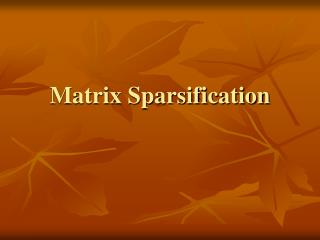# Matrix Sparsification - PowerPoint PPT PresentationDownload PresentationMatrix Sparsification

Matrix Sparsification
Download Presentation## Matrix Sparsification

- - - - - - - - - - - - - - - - - - - - - - - - - - - E N D - - - - - - - - - - - - - - - - - - - - - - - - - - -
##### Presentation Transcript

1. Matrix Sparsification

2. Problem Statement • Reduce the number of 1s in a matrix

3. Measuring Sparsity • The way I measured sparsity was by adding up the total number of 1s in a matrix and dividing by the total number of elements • This gives you a number between 0 and 1 that tells you what percentage of the matrix is filled with ones

4. Experiment Setup • First I generated H, which is a sparse 300x582 matrix with a column weight of 3, sparsity=.01 • Then I multiplied it by a random invertible matrix. • D is the resulting dense matrix, sparsity≈.5 • Then I tried to make D as sparse as the original matrix

5. Comparing rowspaces • From the sparse H matrix, generate a G matrix so that mod(H*G,2)=0 • Then test D and any sparsified versions of D to ensure that mod2 of multiplication by G still results in a 0 matrix

6. GF2 difficulties • Matrix sparsification is difficult in GF2 because it requires a different set of math rules • For example: • With real numbers, the vectors [0 1 1],[1 0 1], and [1 1 0] are independent • In GF2, any one of those vectors can be made from the other two

7. Row Echelon Form • The dense matrix starts with sparsity≈.5 • In an [m x n] matrix, row reduction will give m columns with only one 1 in them. • The rest of the columns should be approximately half 1s • Now sparsity≈(m+.5*(n-m)*m)/(n*m) • The more square a matrix is, the more this step helps.

8. Null Space • Row Echelon Form tries to make the pivot columns the earliest possible columns • By computing the null space of the original matrix, and then computing the null space of that null space, you get back the original rowspace • Now the pivot columns are in different locations

9. SP2 function • Find the row which reduces the number of 1s the most. • A(row,:)*AT is a vector that gives the number of matching 1s in each row (i.e. the number of 1s that will be eliminated if the rows are added) • (1-A(row,:))*AT = a vector giving you the number of 1s that will be introduced if the rows are added.

10. SP2 function • If B= A(row,:)*AT - (1-A(row,:))*AT, then finding the maximum of B will reduce the sparsity the most per row addition. • But first, you have to set B(row)= -n, because otherwise B(row) will always be the maximum, and you can’t add a row to itself.

11. SP2 function • If max(B) is positive, then adding rows helps sparsify the matrix • If max(B) is 0, then adding rows keeps the sparsity the same, but changes the location of the 1s • When max(B) is negative, it makes the matrix more dense, but it can be helpful in overall sparsification because it helps get you out of local minimums

12. SP3 function • First I make a matrix of all possible combinations of two different rows and also the original rows that I’m testing on • This new matrix has (m-1)Choose2 + (m-1) rows • Then I follow the same process as with the SP2 function, but using this new matrix, instead of one made up of only the original rows

13. Why not add 3? • The matrix size grows too quickly. • With 300 rows, the matrix for adding 1 or 2 rows has 44850 rows, and the matrix for adding 1 to 3 rows would have 4455399 rows.

14. Why not add 3? • You could break the matrix down into multiple smaller matrices, then save the best row from each matrix and add in the best overall row • One reason for the significant improvement when adding two rows instead of one is that in GF2 1+1=0.

15. Orthogonal Projection • The easiest way to span a space is with orthogonal vectors • If the projection of vector a onto b is greater than .5, then b is a significant component of a, so removing it will make the vectors closer to orthogonal, and the matrix more sparse • i.e. if((a·b)/(|b|2)>.5) rowa=mod2(rowa+rowb)

16. LDPC Decoding results • In LDPC codes, every 1 in a matrix represents a connection between check nodes and variable nodes. • Reducing the sparsity of a matrix makes LDPC decoding faster, and more reliable

17. SNR Vs. Probability of Error